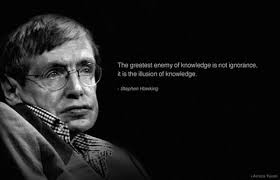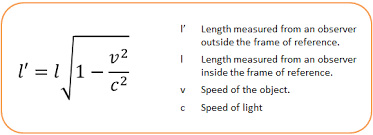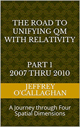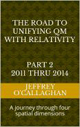# 4 spatial dimensions and A Theory of Everything

One cannot deny that Quantum mechanics, the theory that defines the tiny world of particles and Einstein’s theories, the one that defines what we see through a telescope have been the most successful scientific theories of modern times However, attempts to bring these two theories together and define "A Theory of Everything" have been unsuccessful.

However, the fact that we have been unable to do so suggests that one or both of these theoretical models does not describe the true nature of reality because the world we see through a telescope must have its foundations in the world of the very small therefore they must be connected.There can be many reasons for this.  One is that foundational assumptions of either or both of them is incorrect.  In other words, the world of the tiny may not be governed by probabilities as quantum theory suggests or the world, we see though a telescope may not be ruled by relativistic properties of four dimensional space-time.

However, there is another possibility that many have over looked is that even though their mathematics makes very accurate perditions of experimental observations they do not accurately define reality of their operating environments.

For example, Einstein mathematically defined the physical structure of the universe in terms of the geometry four dimensional space-time.

However, when using the constant velocity of light and the velocity of objects that do not move at that speed to define its geometric properties he provided a way of mathematical converting a unit of time in a space-time universe to unit of space in one physically consisting of only four *spatial* dimensions.

In other words, their is an equally valid interpretation of his mathematics in terms of only four spatial dimensions.

Since both of these solutions that of four dimensional space-time and four spatial dimensions would yield the same numerical results it gives one a different way of connecting his theories to those of Quantum mechanics based on the physical properties of four spatial dimensions instead of four dimensional space-time.

Quantum Theory on the other hand defines tiny world of particles in terms of the non physical probabilities associated with SchrÃ¶dinger wave equation which as mentioned earlier no one has been able to physical connect to the space-time universe define by Einstein.

However, the fact that Einstein provided an alternative solution to his mathematics in terms of four spatial dimensions suggests it may be possible to make that connection and therefore define a Theory of Everything by using the alternative solution of four spatial dimension that his theory provides instead of one based on four dimensional space-time.

For example the article "Why is mass quantized?" Oct. 4, 2007 showed one could derive the quantum mechanical properties of energy in terms of a resonant "system" or structure formed by a energy wave on the surface of a three-dimensional spatial manifold with respect to a fourth *spatial* dimension.

Briefly that article showed the four conditions required for resonance to occur in a three-dimensional environment, an object, or substance with a natural frequency, a forcing function at the same frequency as the natural frequency, the lack of a damping frequency and the ability for the substance to oscillate can be meet in one consisting of terms of four spatial dimension.

Its continuous properties would allow an energy wave on a "surface’ of a three-dimensional space manifold to oscillate with respect to a fourth *spatial* dimension thereby fulfilling one of the requirements for resonance to occur.

These oscillations would be caused by an event such as the decay of a subatomic particle or the shifting of an electron in an atomic orbital. This would force the "surface" of a three-dimensional space manifold with respect to a fourth *spatial* dimension to oscillate with the frequency associated with the energy of that event.

Therefore, these oscillations in a continuous non-quantized field of energy would meet one of the requirements mentioned above for the formation of a resonant system or "structure" in space.

Observations of a three-dimensional environment show the energy associated with resonant system can only take on the incremental or discreet values associated with a fundamental or a harmonic of the fundamental frequency of its environment.

Similarly the energy associated with resonant systems in four *spatial* dimensions could only take on the incremental or discreet values associated a fundamental or a harmonic of the fundamental frequency of its environment.

These resonant systems in a space-time environment would be responsible for the incremental or discreet energy associated with quantum mechanical systems.

Another requirement for a resonate system to be formed is that the wave must be confined to specific volume of space.

However, one can also define the confinement of the resonant component of a particle and therefore establish a physical connection to the wave particle duality quantum mechanics associates with energy in terms of the relativistic properties of four *spatial* dimensions.

In physics, a point on the two-dimensional surface of paper is confined to that surface.  However, that surface can oscillate up or down with respect to three-dimensional space.

Similarly an object occupying a volume of three-dimensional space would be confined to it however, it could, similar to the surface of the paper oscillate "up" or "down" with respect to a fourth *spatial* dimension.

The confinement of the "upward" and "downward" oscillations of a three-dimension volume with respect to a fourth *spatial* dimension is what defines the spatial boundaries associated with a particle in the article "Why is energy/mass quantized?".

In other words, an energy wave in four *spatial* dimensions will maintain its wave properties unless it is confined to three by an observation, therefore it it always be view as a particle when an observation is made and any energy left over from the formation of its resonate structure will be radiating from the point of observation in the form of light or an energy wave.

The physics of wave mechanics also tells us that due to their continuous properties the energy waves the article "Why is energy/mass quantized?" Oct. 4, 2007 associated with a quantum system would be distributed throughout the entire "surface" a three-dimensional space manifold with respect to a fourth *spatial* dimension.

For example the energy of a vibrating or oscillating ball on a rubber diaphragm would be disturbed over its entire surface while the magnitude of those vibrations would decrease as one move away from the focal point of the oscillations.

Similarly if the assumption that quantum properties of energy are a result of vibrations or oscillations in a "surface" of three-dimensional space is correct those oscillations would be distributed over the entire "surface" three-dimensional space while the magnitude of those vibrations would be greatest at the focal point of the oscillations and decreases as one moves away from it.(Some may question the fact that the energy wave associated with particle would be distributed over the entire universe.  However, the relativistic properties of space-time and four spatial dimensions tell that distance perceived by objects or particles in relative motion is dependent on their velocity which become zero at the speed of light.  Therefore, from the perspective of an energy wave moving at the speed of light, the distance between all points in the universe along it velocity vector is zero.  In other words, it’s energy is distributed or simultaneous exists at every point in the universe along its velocity vector.  There can be not other conclusion if one accept the validity of Einstein’s theories.)

As mentioned earlier the article â€œWhy is energy/mass quantized?â€ shown a quantum particle is a result of a resonant structure formed by an energy wave on the "surface" of a three-dimensional space manifold with respect to a fourth *spatial* dimension.

Yet the science of Wave Mechanics tells us resonance would most probably occur on the surface of the rubber sheet were the magnitude of the vibrations is greatest and would diminish as one move away from that point,

Similarly a particle would most probably be found were the magnitude of the vibrations in a "surface" of a three-dimensional space manifold is greatest and would diminish as one move away from that point.

This shows how, by interpreting Einstein space-time theories in their equivalent four spatial dimension one can connect the non physical probabilities associated with SchrÃ¶dinger wave equation to the reality of the world defined by him.Additional it shows, by changing our interpretation of Einstein’s theories from four dimensional space-time to it equivalent in four spatial dimensions allows one to clearly understand the physical connection between the probabilistic world of quantum theory and the relativistic world of his theories, thereby allowing one to form a Theory of Everything.

Later Jeff

 The Road to Unifying QM with Relativity part 1 2007 thru 2010Ebook \$8.00Paper Back \$15.00 The Road to Unifying QM with Relativity part 2 2011 thru 2014Ebook \$8.00Paper Back \$16.00 The Road to Unifying QM with Relativity part 3 2015 thru 2020Ebook \$8.00Paper Back \$18.00